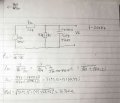# Resistor and capacitor in parallel

#### Morten Andersen

Joined Jun 7, 2019
25
Maybe a pretty basic question, but never the less, im in doubt.
To find an equivalent of an resistor parallel with an capacitor. Is the way to do it that you take the reaktens of the capacitor parallel with the resistor. so (R^-1+(1/2*pi*f*c)^-1)^-1 or in other words R||XC?

#### dl324

Joined Mar 30, 2015
12,238
You are missing a set of parenthesis around 2*pi*f*C.

Using tex tags would give a more readable equation:

This is what you wrote:
(R^-1+(1/2*pi*f*c)^-1)^-1
$$\frac{1}{\frac{1}{R}+\frac{πfC}{2}}$$

This is what you meant to write:
$$\frac{1}{\frac{1}{R}+\frac{1}{2πfC}}$$

•atferrari

#### MrAl

Joined Jun 17, 2014
7,884
Hi,

Actually you have to do this:
Z=1/(1/ZR+1/ZC)
where
ZR=R
ZC=1/(s*C) or 1/(j*w*C)
and of course w=2*pi*f.

This means that Z is a complex quantity with both magnitude and phase angle.
See if you can calculate Z now.

Do you understand this or no?

Last edited:
•Morten Andersen

#### Morten Andersen

Joined Jun 7, 2019
25
Oh yea, thoose parentheses slipped, that was what I meant.

I understand that the impedance of a capacitor is a complex number.

But let's say I'm to do a voltage division between a resistor R1 and a resistor R2 parallel with condensate C, and I dont care about the angle. Then is it correct to just see R2 as a parallel to C and use the formula for 2 parallels, with just Xc being 1/(2*pi*f*C)?

#### MrAl

Joined Jun 17, 2014
7,884
Hello again,

Well let's see...

The impedance of the cap is:
ZC=1/(j*2*pi*f*C)

the reactance of the cap is:
XC=1/(2*pi*f*C)

Now a voltage divider with resistor on top and cap on bottom using XC is:
Vo1=Vin*XC/(XC+R)=Vin/(2*pi*f*C*R+1)

and the same voltage divider using ZC is:
Vo2=Vin*ZC/(ZC+R)=-(j*Vin)/(2*pi*f*C*R-j)=Vin/(4*pi^2*f^2*C^2*R^2+1)-(2*j*pi*f*Vin*C*R)/(4*pi^2*f^2*C^2*R^2+1)
and finding the magnitude we get:
|Vo2|=abs(Vin)/sqrt(4*pi^2*f^2*C^2*R^2+1)

Now substitute values R=1, C=2, f=3, Vin=4 we get:
Vo1=0.1033615452525 volts
Vo2=0.10606598691681 volts

but now substitute values R=1, C=1, f=1, Vin=4 we get:
Vo1=0.54921024679365 volts
Vo2=0.62870690191036 volts

So in one case we got pretty close but in the other case we got a significant difference.
So in general we have to say that we can not use the reactance of the cap we should use the impedance and do the full calculation.
I am guessing that we could come up with some rule like if the frequency is high compared to the reciprocal of the RC time constant we get close but if not we get a larger error but i havent investigated this in detail, there may be some other things to factor in.

I would suggest you try this yourself to prove it to yourself.
Try R=4,C=3,f=2,Vin=1 for example.

•Morten Andersen

#### Morten Andersen

Joined Jun 7, 2019
25
My only confusion was if I was able to do calculations in the circuit using the reactance instead of the impedance. But I can understand that I can't. Thank you very much.

#### MrAl

Joined Jun 17, 2014
7,884
My only confusion was if I was able to do calculations in the circuit using the reactance instead of the impedance. But I can understand that I can't. Thank you very much.
Hi again,

Well the max error factor for one method divided by the other is 1/sqrt(2) whcih is about 0.7071 so the max error is about 140 percent. That means if you get a result of around 0.7 with the reactance method it could really be closer to 1.0 or so doing the full calculation. So a result of 7 volts with the reactance method could really be as high as 10 volts but that's the max.

•Morten Andersen

#### Morten Andersen

Joined Jun 7, 2019
25
Does this look about right ?

#### MrAl

Joined Jun 17, 2014
7,884
Does this look about right ?
Yes that looks right but only for the parallel combination you are showing.
To get the entire network you first add the complex parallel combination to the other resistor value then find the magnitude. But yes that would be right for the parallel combo alone.

Here is a better drawing yours was kind of hard to see on my monitor.•Morten Andersen

#### Morten Andersen

Joined Jun 7, 2019
25
Ah okay, so I do complex calculations for the voltage divider aswell, and then find the magnitude of Vout. I'll get it!
The is actually part of a larger assignment containing a small signal equivalent of a MOSFET amplifier circuit - I have just been stuck on this part.

#### Morten Andersen

Joined Jun 7, 2019
25
So the result ends up like this - does it look right?

#### MrAl

Joined Jun 17, 2014
7,884
Hi,

Yes 0.0038 is correct to that many digits.
I get 0.00379373 using six non zero digits so 0.0038 would be that rounded.

•Morten Andersen